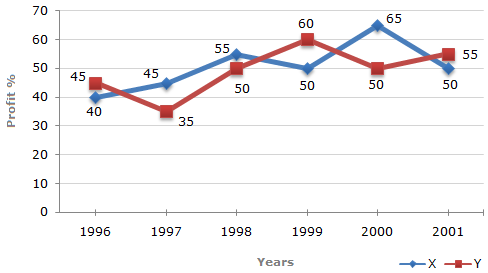# Data Interpretation - Line Charts - Discussion

Discussion Forum : Line Charts - Line Chart 4 (Q.No. 1)
Directions to Solve

The following line graph gives the percent profit earned by two Companies X and Y during the period 1996 - 2001.

Percentage profit earned by Two Companies X and Y over the Given Years

 %Profit = Income - Expenditure x 100 Expenditure1.
The incomes of two Companies X and Y in 2000 were in the ratio of 3:4 respectively. What was the respective ratio of their expenditures in 2000 ?
7:22
14:19
15:22
27:35
Explanation:

Let the incomes in 2000 of Companies X and Y be 3x and 4x respectively.

And let the expenditures in 2000 of Companies X and Y be E1 and E2 respectively.

Then, for Company X we have:

 65 = 3x - E1 x 10065 = 3x - 1E1 = 3x x100.... (i) E1 100 E1 165

For Company Y we have:

 50 = 4x - E2 x 10050 = 4x - 1E2 = 4x x100.... (ii) E2 100 E2 150

From (i) and (ii), we get:

E1 =
 3x x100165
= 3 x 150 = 15 (Required ratio).
E2
 4x x100150
4 x 165 22

Discussion:
15 comments Page 1 of 2.

Karma Tsh said:   2 years ago
@Lavanya;

450/660 = 15:22.
Ways:
450/15=30,
So 30*22 = 660.

Pema said:   5 years ago
How the calculation got 3x*100÷165? Explain me in detail.

Lavanya said:   6 years ago
@Prachi.

450/660=15:22?

AMBER SINGH said:   6 years ago
1 is added to 65/100 which then gives us 165/100.

Tamilarasan said:   7 years ago
@All.

Taking out the expenditure common the fraction becomes as I/E -1.

Twinkle said:   7 years ago
Yes, same doubt for me. Where does the - 1 goes?

Raja naidu said:   7 years ago
Where did that -1 go?

Abhi said:   8 years ago
3x = 165.
4x = 150.

3x/165*150/4x = 15/22.

Tinie said:   8 years ago
Why am I getting 15/44 --- 3/4*150/165 --- 3/4*30/33 --- 3/4*10/11 --- 30/44?

Prachi said:   9 years ago